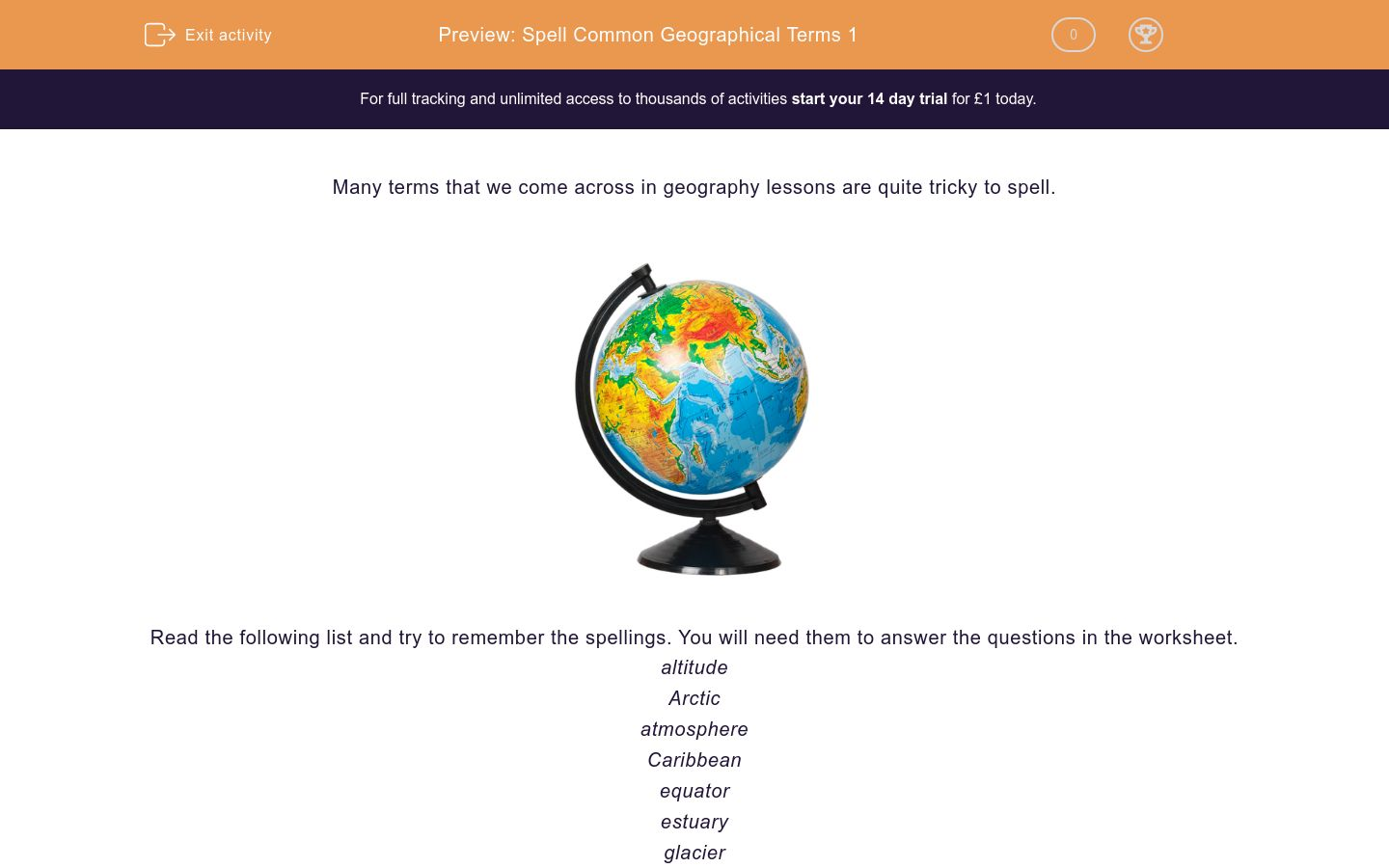# Spell Common Geographical Terms 1

In this worksheet, students practise spelling geographical terms that often prove tricky.Key stage:  KS 3

Curriculum topic:   Writing

Curriculum subtopic:   Use Accurate Grammar, Punctuation and Spelling

Difficulty level:### QUESTION 1 of 10

Many terms that we come across in geography lessons are quite tricky to spell.Read the following list and try to remember the spellings. You will need them to answer the questions in the worksheet.

altitude

Arctic

atmosphere

Caribbean

equator

estuary

glacier

ocean

plateau

vegetation

Below is a definition of one of the words listed in the introduction. Write the word in the answer box.

an imaginary line drawn around the earth, at equal distance from the north and south poles

Below is a definition of one of the words listed in the introduction. Write the word in the answer box.

One of the large areas of saltwater that cover most of the Earth.

Below is a definition of one of the words listed in the introduction. Write the word in the answer box.

A region between North and South America, including a portion of the West Indian islands.

Below is a definition of one of the words listed in the introduction. Write the word in the answer box.

an area of fairly level high ground

Below is a definition of one of the words listed in the introduction. Write the word in the answer box.

the plants of a particular region or habitat

Below is a definition of one of the words listed in the introduction. Write the word in the answer box.

the height of an area above sea level

Below is a definition of one of the words listed in the introduction. Write the word in the answer box.

the tidal mouth of a river

Below is a definition of one of the words listed in the introduction. Write the word in the answer box.

a large body of ice moving slowly down a slope or valley

Below is a definition of one of the words listed in the introduction. Write the word in the answer box.

the mass of air surrounding the planet

Below is a definition of one of the words listed in the introduction. Write the word in the answer box.

The region around the north pole.

• Question 1

Below is a definition of one of the words listed in the introduction. Write the word in the answer box.

an imaginary line drawn around the earth, at equal distance from the north and south poles

equator
the equator
EDDIE SAYS
Remember that the ending is -or and not -er.
• Question 2

Below is a definition of one of the words listed in the introduction. Write the word in the answer box.

One of the large areas of saltwater that cover most of the Earth.

ocean
EDDIE SAYS
The 'sh' sound is represented by 'ce'.
• Question 3

Below is a definition of one of the words listed in the introduction. Write the word in the answer box.

A region between North and South America, including a portion of the West Indian islands.

Caribbean
the Caribbean
EDDIE SAYS
People often forget the second 'b' in this word.
• Question 4

Below is a definition of one of the words listed in the introduction. Write the word in the answer box.

an area of fairly level high ground

plateau
EDDIE SAYS
This word comes from French, which is why it ends in the letters -eau but rhymes with 'window'.
• Question 5

Below is a definition of one of the words listed in the introduction. Write the word in the answer box.

the plants of a particular region or habitat

vegetation
EDDIE SAYS
The second 'e' in this word is the tricky part. People often write 'vegitation'.
• Question 6

Below is a definition of one of the words listed in the introduction. Write the word in the answer box.

the height of an area above sea level

altitude
EDDIE SAYS
People often pronounce this word as if it has 'ch' in the middle.
• Question 7

Below is a definition of one of the words listed in the introduction. Write the word in the answer box.

the tidal mouth of a river

estuary
EDDIE SAYS
People often forget the 'a' in this one.
• Question 8

Below is a definition of one of the words listed in the introduction. Write the word in the answer box.

a large body of ice moving slowly down a slope or valley

glacier
EDDIE SAYS
This word can be pronounced with a long 'ai' sound or a short 'a' sound.
• Question 9

Below is a definition of one of the words listed in the introduction. Write the word in the answer box.

the mass of air surrounding the planet

atmosphere
the atmosphere
EDDIE SAYS
Watch the 'ph' in this one!
• Question 10

Below is a definition of one of the words listed in the introduction. Write the word in the answer box.

The region around the north pole.

Arctic
the Arctic
EDDIE SAYS
It is easy to forget the middle 'c' in this word.
---- OR ----

Sign up for a £1 trial so you can track and measure your child's progress on this activity.

### What is EdPlace?

We're your National Curriculum aligned online education content provider helping each child succeed in English, maths and science from year 1 to GCSE. With an EdPlace account you’ll be able to track and measure progress, helping each child achieve their best. We build confidence and attainment by personalising each child’s learning at a level that suits them.

Get started